# KSEEB SSLC Class 10 Maths Solutions Chapter 9 Polynomials Ex 9.1

KSEEB SSLC Class 10 Maths Solutions Chapter 9 Polynomials Ex 9.1 are part of KSEEB SSLC Class 10 Maths Solutions. Here we have given Karnataka SSLC Class 10 Maths Solutions Chapter 9 Polynomials Exercise 9.1.

## Karnataka SSLC Class 10 Maths Solutions Chapter 9 Polynomials Exercise 9.1

Question 1.
The graph of y = p(x) are given in the following figure, for some polynomials p(x). Find the number of zeros of p(x) in each case.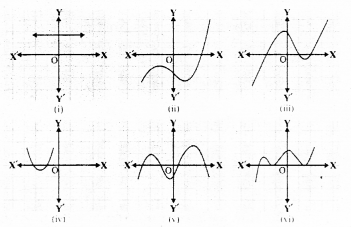Solution:
(i) Graph of y = p(x) will not intersects x-axis.
∴ No zero for this polynomial.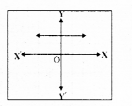(ii) Graph of y = p(x) intersects x-axis at only one point.
Only one zero is there for this polynomial.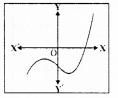(iii) y = p(x) intersects x-axis at 3 points.
∴ It has 3 zeroes.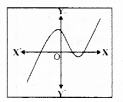(iv) y = p(x) intersects x-axis at 2 points.
∴ Two zeroes for this polynomial.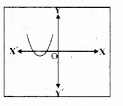(v) y = p(x) intersects x-axis at four (4) points.
∴ Four zeroes for this polynomial.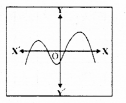(vi) y = p(x) intersects x-axis at three (3) points.
∴ This polynomial has 3 zeroes.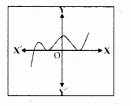We hope the given KSEEB SSLC Class 10 Maths Solutions Chapter 9 Polynomials Ex 9.1 will help you. If you have any query regarding Karnataka SSLC Class 10 Maths Solutions Chapter 9 Polynomials Exercise 9.1, drop a comment below and we will get back to you at the earliest.